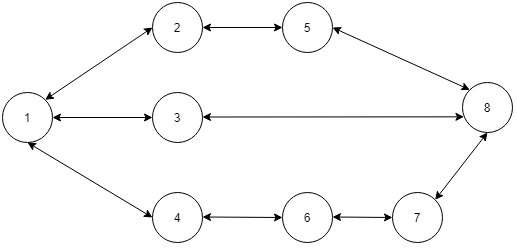# Shortest path in an unweighted graph

Posted: 18 Dec, 2020
Difficulty: Moderate

## PROBLEM STATEMENT

#### A path between house ‘S’ to house ‘T’ is defined as a sequence of vertices from ‘S’ to ‘T’. Where starting house is ‘S’ and the ending house is ‘T’ and there is a road connecting two consecutive houses. Basically, the path looks like this: (S , h1 , h2 , h3 , ... T). you have to find the shortest path from ‘S’ to ‘T’.

##### For example
``````In the below map of Ninjaland let say you want to go from S=1 to T=8, the shortest path is (1, 3, 8). You can also go from S=1 to T=8  via (1, 2, 5, 8)  or (1, 4, 6, 7, 8) but these paths are not shortest.
``````##### Input Format :
``````The first line of input will have a single positive integer ‘T’, denoting the number of test cases.

The first line of each test case has two positive integers ‘N’ and ‘M’ denoting the number of houses and the number of roads in the ninja land.

The second line contains two integers ‘S’ and ‘T’ denoting the starting and ending house in the path.

Next M lines each contain two integers ‘X’ and ‘Y’. Which denotes a road between house ‘X’ and house ‘Y’.
``````
##### Output Format :
``````You have to return a vector of nodes denoting the shortest path starting from ‘S’ and ending in ‘T’.

If there is more than one shortest path you can return any one of them.

The output of each test case will be "Correct" if you have returned the correct answer, else it will be "Incorrect".

Print the output of each test case in a separate line.
``````
##### Note :
``````You do not need to print anything, it has already been taken care of. Just implement the given function.
``````
##### Constraints :
``````1 <= T <= 100
2 <= N <= 10 ^ 3
1 <= M <= min( N *(N - 1) / 2 , 1000 )
1 <= S, T <= N

Time Limit: 1 sec
``````Approach 1
• Store all the edges in the form of an adjacency list ADJ. if ADJ[X][j] = Y which means there is an edge from X to Y.
• Declare a queue Q and push S in it and also declare two vectors VISITED and DISTANCE which will store whether a house is visited or not and what is a distance of the house from house S respectively.
• We will also store the PARENT, that store the node from which we will reach the current node. It will help us in building the path afterwards.
• Mark S as visited and set DISTANCE[S] = 0
• Do the following operations until the Q is not empty.
• Select a vertex which is at the front end of the queue let's say it X. remove it from the queue.
• Iterate to all the neighbouring vertices of X using ADJ. let’s say it Y and if Y is unvisited.
• push Y in the Q.
• Mark Y as visited.
• Set DISTANCE[Y] = DISTANCE[X]+1
• Make the PARENT[Y] = X
• Once we do this we will get the minimum distance of all vertices from S and now we only need to rebuild the path. For this, we can take a vector PATH and push T in it and mark CUR=T.
• Do the following until CUR not equal to S
• CUR =  PARENT[CUR]
• Add CUR into the PATH
• By this, we will trace the path in the reverse direction.
• By doing this we have recreated the path now just reverse the PATH and return it.
• Let us take an example of the following graph.
• n=4 , m=4
• 1 2
• 1 3
• 2 3
• 3 4
• Here we have S = 1 and T = 4, so we start our bfs with node 1
• In the first iteration, we have node 1, we will add its neighbours that is 2 and 3 in the queue and set distance of 2 and 3 equal to 2;
• Now in the second iteration, we have 2 and there are no unvisited neighbours of 2 so we will move on.
• In third iteration we have and there is only one neighbour that is unvisited that is 4 so we will set the distance of 4 equal to 3
• we backtrack and we get a path ( 1, 2, 3 )